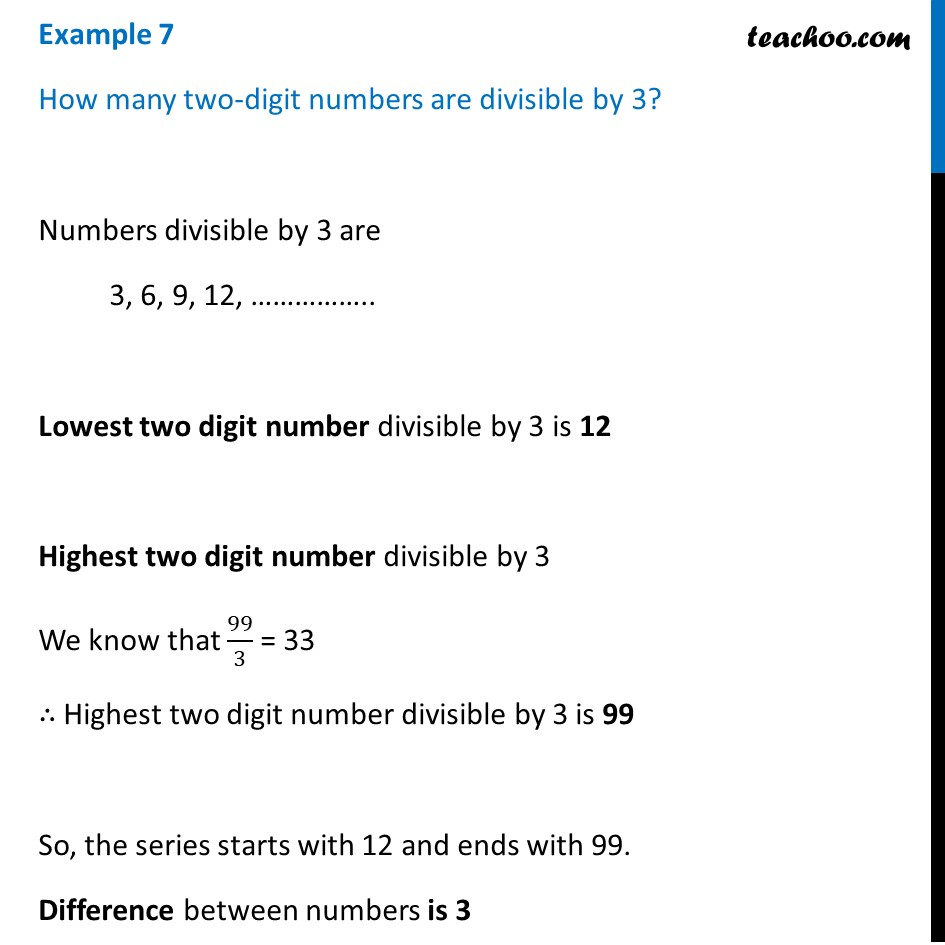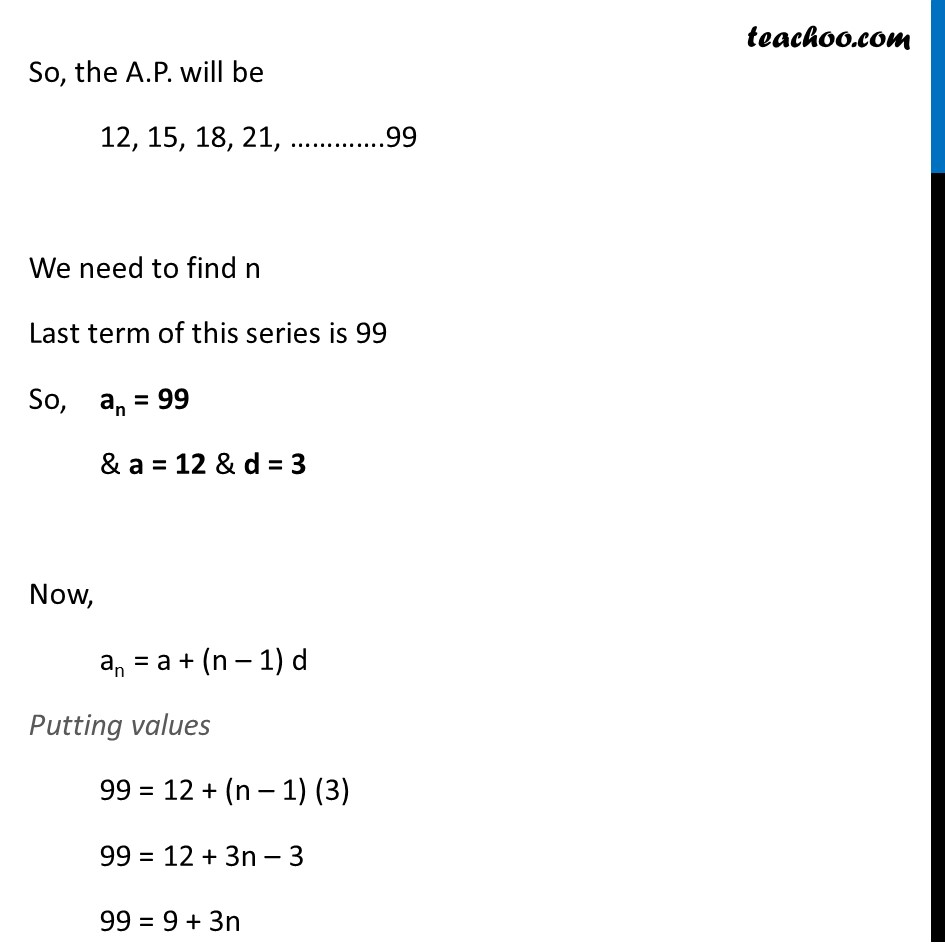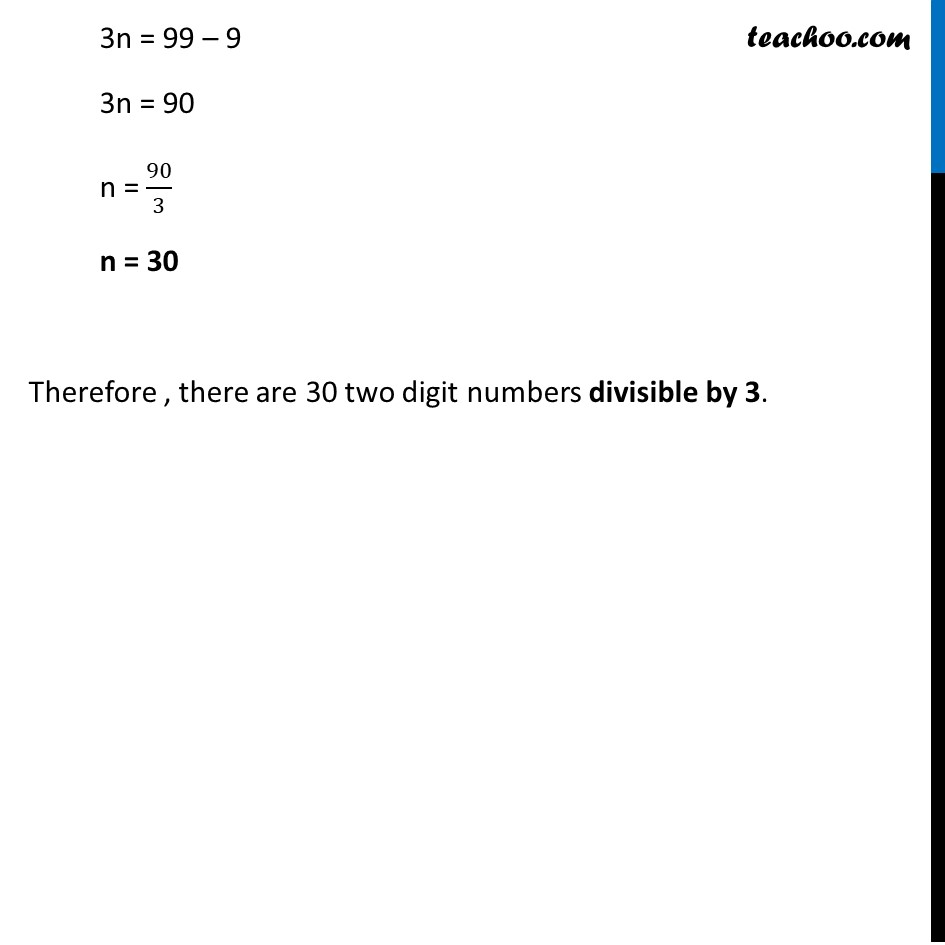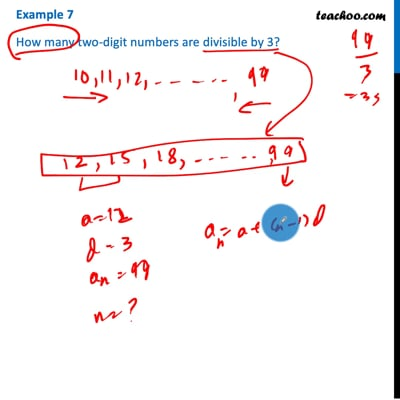Divisible/ multiples

Chapter 5 Class 10 Arithmetic Progressions
Concept wiseThis video is only available for Teachoo black users

Introducing your new favourite teacher - Teachoo Black, at only ₹83 per month

### Transcript

Example 7 How many two-digit numbers are divisible by 3? Numbers divisible by 3 are 3, 6, 9, 12, …………….. Lowest two digit number divisible by 3 is 12 Highest two digit number divisible by 3 We know that 99/3 = 33 ∴ Highest two digit number divisible by 3 is 99 So, the series starts with 12 and ends with 99. Difference between numbers is 3 So, the A.P. will be 12, 15, 18, 21, ………….99 We need to find n Last term of this series is 99 So, an = 99 & a = 12 & d = 3 Now, an = a + (n – 1) d Putting values 99 = 12 + (n – 1) (3) 99 = 12 + 3n – 3 99 = 9 + 3n 3n = 99 – 9 3n = 90 n = 90/3 n = 30 Therefore , there are 30 two digit numbers divisible by 3.